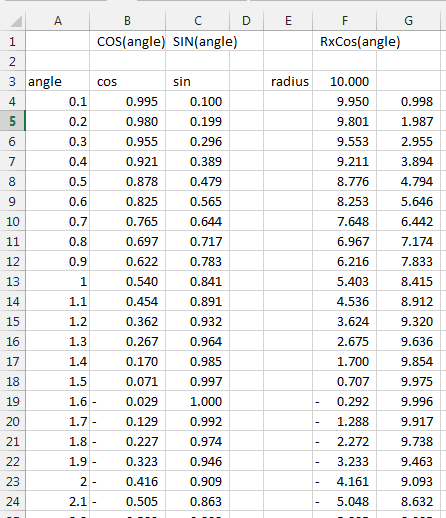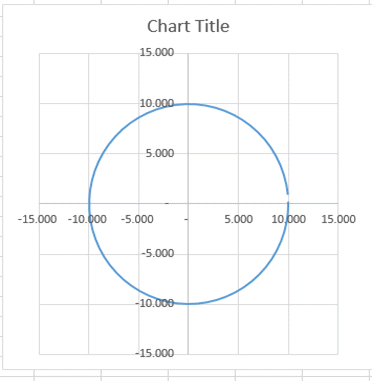## Example: Draw a circle in Microsoft Excel

You are wondering how to draw a circle. Here it is shown.

The first thing to do is to understand that a circle is made can be created using two ways.

1) a parametric equation x^2 + y^2 = R^2  where R is the radius and X and Y are the coordinates of each point.

2) the sin (stands for sinus) and cos (stands for cosinus) function. x = R x Cos(angle) and y = R x Sin(angle)

The easiest is the second one. By using the sinus and cosinus in Excel you can draw a perfect circle.

So lets create a table wit hthe angle, X and Y and draw it.And here the chart (SCATTER CHART) of the column B and C or F and G. Depends if you want a radius of 10 or 1.Et Voilà  Here you have a circle done with the Cos and Sin function of Excel.